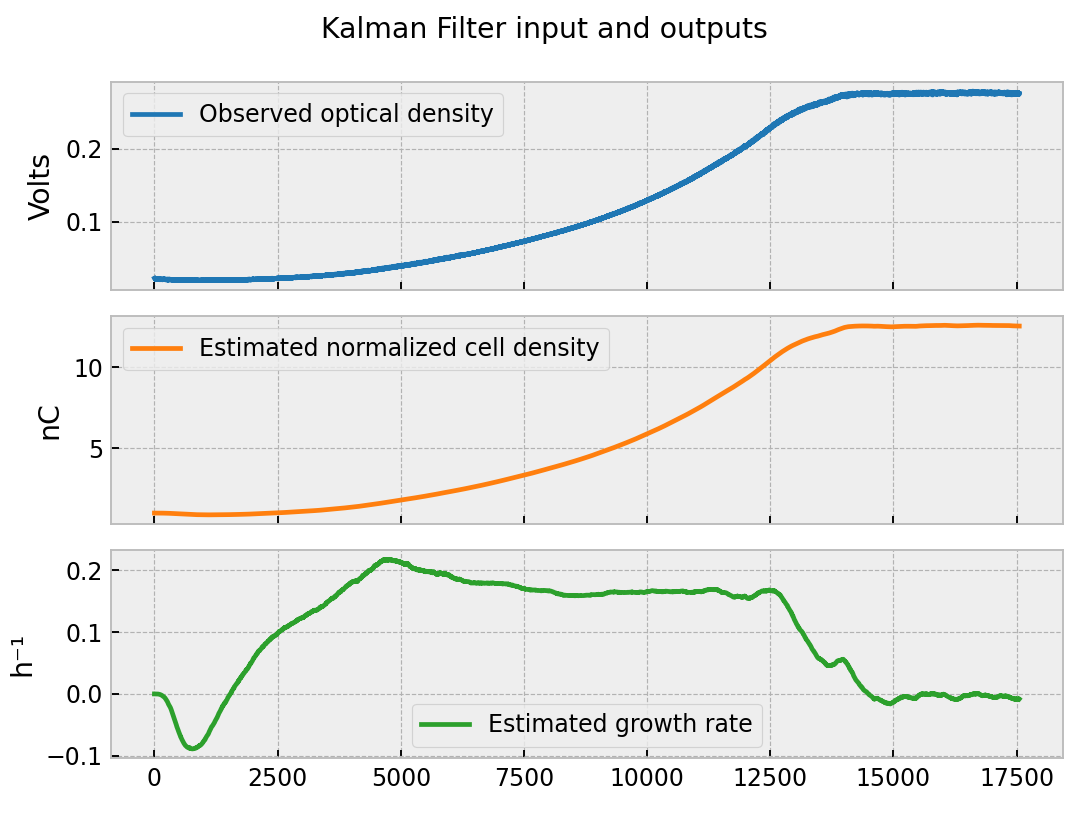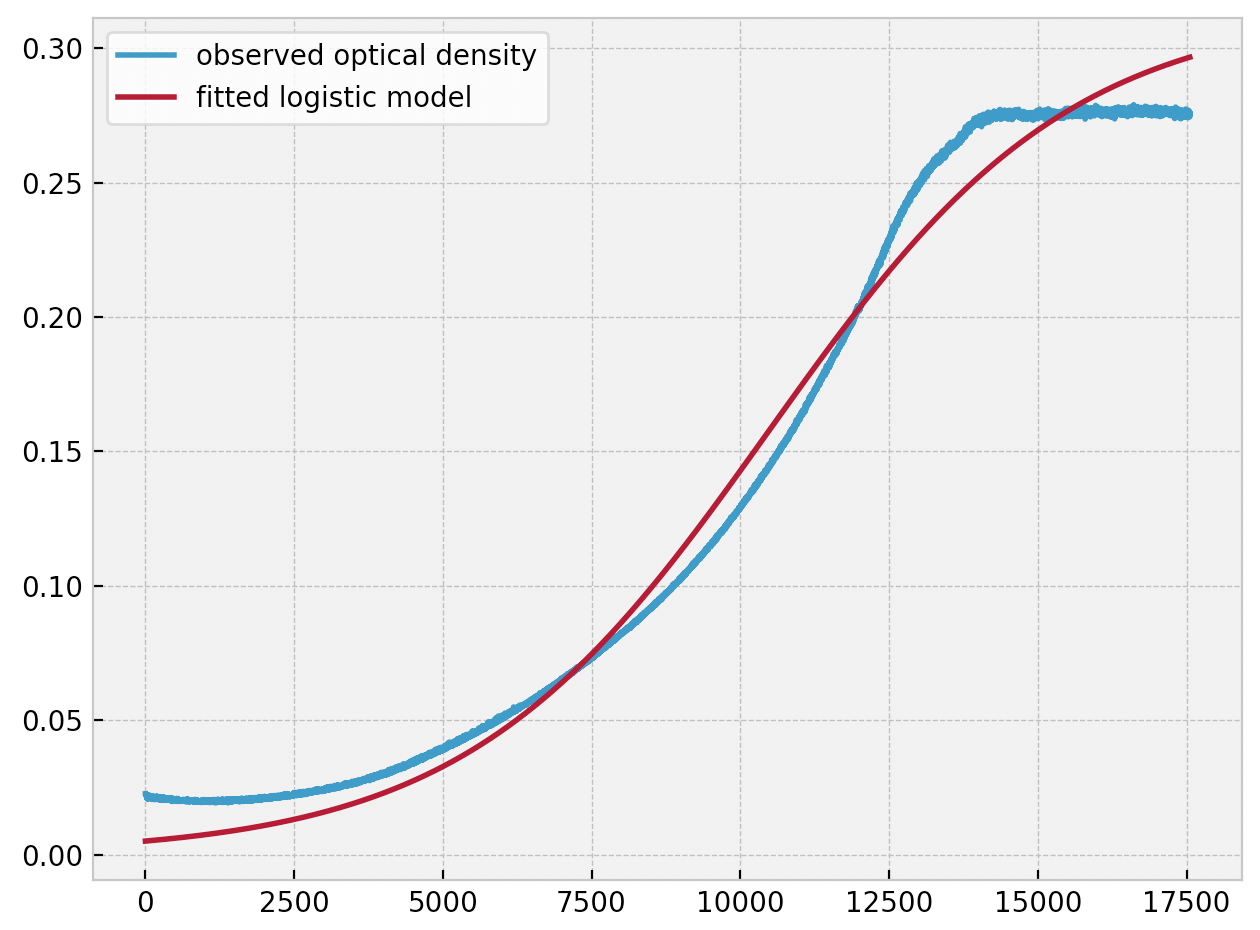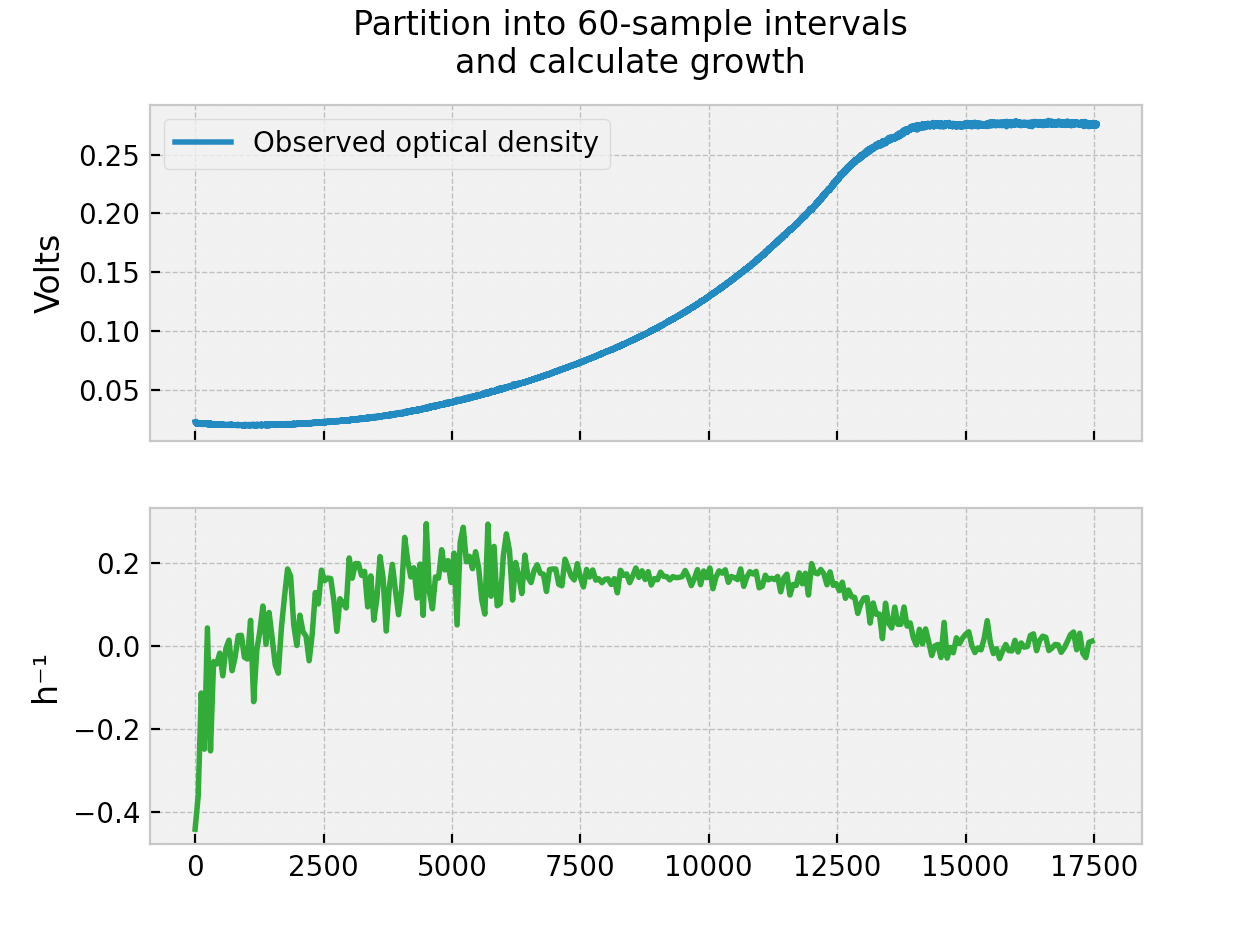# Pioreactor development log #5

⭐️ One of our goals with the Pioreactor is design it such that you don't need to be a biologist,  or an electrical engineer, or relevant for this article: a statistician. This article describes our internal algorithm that computes the culture's growth rate, but importantly: you don't need to know this algorithm to use the Pioreactor! We've designed the internal statistical algorithm to be robust enough that you can sit back and watch. This article is for the users who really want to dig deep into how we compute growth rates and the statistics behind it.

### Introduction to cell density, optical density, and growth rate.

An important metric in bio-processing is the cell density, or biomass, of a bioreactor. The cell density can be measured directly, but the cost of this is very high. Either you are pulling a sample out and counting cells (slow, manual, and noisy), or passing liquid through a flow cytometer (expensive). A common proxy for cell density is optical density: measuring the amount of light scattering off cells. This approach is fast and inexpensive, but has drawbacks, too:

1. Optical density sensors have upper and lower thresholds: too few cells can be difficult to detect, and too many cells can saturate the sensors (multiple scattering events are unpredictable).
2. Changes in the size of cells during metabolic shifts can change the scattering, hence change optical density measurement.
3. Optical density is unit-less, whereas cell density has units. A calibration curve is needed to convert optical density measurements to cell density (culture specific, too).
4. Non-viable (dead) cells contribute to optical density.
5. Optical density is noisy measurement of cell density. That is, for any true cell density, the optical density will have some statistical standard error that can't be reduced.

Suffice to say, optical density ≠ cell density, but tuned correctly, optical density has a very high correlation to cell density and is often good enough. This is why optical density is used so commonly in microbiology.

The Pioreactor, our affordable bioreactor, uses optical density to measure cell density. In fact, we have multiple optical density sensors. We call measurements from these sensors our raw optical density measurement, in volts. Taking a measurement is cheap, so we record a new measurement every 5 seconds. Because of the variability in each Pioreactor, the raw optical density measurements will vary between Pioreactor, and they don't have a direct interpretation anyways. For example, what does a raw optical density of 0.4341 V mean? Is that high, low, neither? So we don't normally expose the raw optical density to users.

Instead, we will investigate the normalized optical density defined as the current raw optical density divided by the mean of the raw optical density at the start of the experiment. That is, if $$m_{i, t}$$ is the raw optical density measurement from sensor $$i$$ at time $$t$$, then the normalized OD is defined:

$$\text{nOD}_{i, t} = \frac{m_{i, t}}{\hat{m}_{i, 0:30}}$$

Analogously, if $$C_t$$ is the true cell density at time $$t$$, then the normalized cell density is:

$$\text{nC}_t = \frac{C_t}{\hat{C}_{0:30}}$$

This quantity is interpreted as the excess new cell density (new biomass) created, relative to the initial cell density (biomass). Of course, we don't know this, but we wish to estimate this.

We are also interested in the rate of change of the culture. In fact, as microbes grow exponentially, we are interested in the growth rate, $$r_t$$, as defined by:

$$\text{nC}_{t+Δt} = \text{nC}_t \exp{\left(r_t Δt\right)}$$

This is kinda like the derivative from calculus, but there's an exponential in there (because we know our culture grows exponentially, this mathematical form makes more sense. A growth rate of 0 means no growth, a positive growth rate means exponential growth, a negative growth rate means exponential decay). In fact, this is equivalent to $$\text{nC}_t =\exp{\left(\int_o^t r_s ds\right)}$$. The growth rate, $$r_t$$, has units that are defined by the time step. In our case, we use the common convention of $$h^{-1}$$.

Note that using normalized cell density doesn't muddy the definition of our growth rate. The quantity $$\hat{C}_{0:30}$$ is present on both sides of the equation above, so whether we use normalized cell density or cell density doesn't matter: we get the same growth rate.

### Estimation

Now that the definitions are present - how do we go about estimating these quantities, specifically the growth rate? Recall we don't have the cell density, so the best we can do is use the optical density measurements as a proxy. I've seen a few ways to estimate the growth rate, and I'll explain why I think they are problematic.

### Parametric model approach

The first option is to fit the entire raw optical density curve to a logistic/Gompertz curve (see Figure 1.). This fitted parametric model of optical density provides us with interpretable parameters. However, this procedure has many problems:

1. Fitting to the parametric model only makes sense after the experiment is completed. So you won't be able to get growth rate measurements in real time.
2. Parametric models are rigid. See Figure 1. for an example of a poor fit. Even worse, if your culture exhibits diauxic growth (two or more growth spurts), they won't neatly fit into any simple "box" provided by parametric models.
3. They provide a single estimate of growth rate, when in fact, growth rate changes over time due to metabolic changes in the culture. We should have a sequence of growth rates over time - not a single value.Figure 1. Fitting a logistic curve to our optical density data from a Pioreactor. Note the very poor fit.

### Partitioning samples approach

To address the problems above, we can try something as follows: take a snapshot of the last $$N$$ optical density measurements, and "locally" the curve looks exponential, so fit the N data points to the model $$A \exp{\left( r_i t \right)}$$. The sequence of $$r_i$$ is the non-parametric growth rate. This is what many research articles do, but there are problems with this, too:

1. How many points, $$N$$, is best? A small $$N$$ means higher resolution growth rate sequence, but also higher estimation error which causes a noisy growth rate sequence. A high $$N$$ is robust to outliers, but also is a lagging measure.
2. The procedure is not recursive, that is, it doesn't borrow relevant information from the past. If we estimate $$r_i$$ in the previous step, we know $$r_{i+1}$$ is going to be close to that estimate. However, we totally ignore all previous estimates (and data points!) in the estimation of the current step.
3. Has a different time period than the original signal. For every $$N$$ optical density measurements, we only observe a single growth rate measurement.Figure 2. The method of looking back $$N=60$$ optical density points, and fitting an exponential curve to them. Then reporting the growth rate as a sequence. This is still noisy, but does provide some shape of what the growth curve might look like.

### Pioreactor's approach: Kalman Filters

Okay, now that I've torn down the existing methods, what method do we implement in Pioreactor? It's a process-based model that uses a Kalman Filter. That is, we model the entire system of unknowns and their relationships, and let statistics handle the estimation. We start by supposing that we know the normalized cell density at time $$t$$, $$\text{nC}_t$$. Given this, we can can guess what the normalized optical density from sensor $$i$$ will be in the next time step (5 seconds in our case):

$$\text{nOD}_{i, t + Δt} = f_i(\text{nC}_t) + \sigma_{\text{obs_std}_i} \cdot \text{noise}$$

where $$f_i$$ can model things like sensor saturation - something the previous estimation methods couldn't do. For our current purposes, we can suppose that our hardware and optical systems are designed well and $$f_i$$ is the identity function. The noise term, and associated coefficient, are systematic noise that we can't avoid.

Given we know $$\text{nC}_t$$, we can also write down how $$\text{nC}_t$$ evolves over time. That's just the growth rate equation from above, with added noise:

$$\text{nC}_{t+Δt} = \text{nC}_t \exp{\left(r_t Δt\right)} + \sigma_{\text{od_std}} \cdot \text{noise}$$

We next write down how we think $$r_t$$ will evolve over time, that is, how might $$r_t$$ relate to $$r_{t+Δt}$$? We have some creativity here, but I propose the following based on my domain knowledge of microbes: i) we assume that the growth rate is very close to the previous time step's growth rate, ii) we assume that there is heterogeneity in how quickly microbes in a culture respond to change, hence a growth rate that is increasing tends to continue to increase, and vice versa. This is modeled by including what we call an "acceleration" term.

$$r_{t+Δt} = r_t + \text{acc}_t Δ t + \sigma_{\text{rate_std}} \cdot \text{noise}$$

We also need to model how that acceleration term evolves. To keep it simple, we assume it is close to its previous value:

$$\text{acc}_{t+Δt} = \text{acc}_t + \sigma_{\text{acc_std}} \cdot \text{noise}$$

What have we done? We have modeled the physical system's variables (observed and hidden) and the relationships between these variables, considering our domain knowledge. Next, with this model in hand, we can pass it to a Kalman Filter for estimation. So when we feed the Kalman Filter the observations $$\text{nOD}_{i, t}$$, we "turn the crank" and output estimates of the hidden (state) variables $$\left[ \text{nC}_t, r_t, a_t \right]$$. This entire procedure provides us with real-time, high resolution, and low-noise estimates of all variables of interest.

Let's see it in action! Figure 3. reports the output from the same optical density data as used above.Figure 3. The results of the Kalman Filter approach. We have a high resolution, low-noise growth rate, and even model the cell-density directly. Note the growth rate curve is a similar shape to the growth rate curve in Figure 2.

### Tuning the parameters of the Kalman Filter

The most annoying part of using Kalman Filters is choosing appropriate entries for the covariance matrices ($$\mathbf{P}$$ and $$\mathbf{Q}$$). This is probably the weakest part of the Kalman Filter approach, but we've done enough experiments that we have provided good defaults in the software.

### Constructing the observed covariance matrix, $$\mathbf{P}$$

Let's start with the easier case of $$\mathbf{P}$$. This covariance matrix contains the information about the noise between the observed variables and the state variables. In our system, this is the variable(s) $$\sigma_{\text{obs_std}_i}$$. To solve this, when we start our experiment, along with calculating the mean of the first 30 samples, $$\hat{m}_{i, 0:30}$$, we also calculate the variance of those samples. We can use this when we calculating the variance of $$\text{nOD_{i,t}}$$:

$$\text{Var}(\text{nOD}_{i,t}) = \text{Var}\left( \frac{ m_{i, 0:30}} {\hat{m}_{i, 0:30}} \right) = \frac{ \text{Var}(m_{i, 0:30})} {(\hat{m}_{i, 0:30})^2}$$

This gives us a pretty good estimate of $$\sigma_{\text{obs_std}_i}$$. However, actually multiply this by a value of 1.0 to 2.0, as we feel this gives a smoother output, and protects better against large outliers. Call this additional factor $$k_{\text{obs_std}}$$, which can be tweaked in the Pioreactor's configuration.

Note: a high value here means that we require a stronger (more extreme) "signal" from the incoming data to get past this filter. It's kinda like a high-pass filter...

So our $$\mathbf{P}$$ matrix for $$n$$ different sensors looks like:

$$\begin{bmatrix} k_{\text{obs_std}}\text{Var}(\text{nOD}_{1,t}) & 0 & 0 \\ ... & ... & ... \\ 0 & 0 & k_{\text{obs_std}}\text{Var}(\text{nOD}_{n,t}) \end{bmatrix}$$

Note the 0s in the off-diagonal. This is telling the Kalman Filter that our signals are independent. Is this true? Not totally, as we do observe some correlation. Another improvement on this is to also calculate the covariance between the signals at the start of the experiment and populate the off-diagonals.

### Constructing the process covariance matrix, $$\mathbf{Q}$$

If you were hoping for a lesson on how to estimate $$\mathbf{Q}$$, I don't have that for you. Luckily, we have only three entries in this matrix of interest (the diagonals of this 3x3 matrix). We perform simulations and vary these entries to find "good" values, which is something we've done. These are editable in the Pioreactor's configuration.

### Conclusion

We hope this blog article gave you more confidence in how we do our internal inference. I think this Kalman Filter is one of our most powerful features. Accurate, real-time growth rates are critical for protocols like the morbidostat and more. We haven't touched on it (yet), but there's also the "trick" to gracefully handle dilution events (which drops the cell density and optical density). The other estimation approaches above can't handle this, but the Kalman Filter can. More on that in the future!